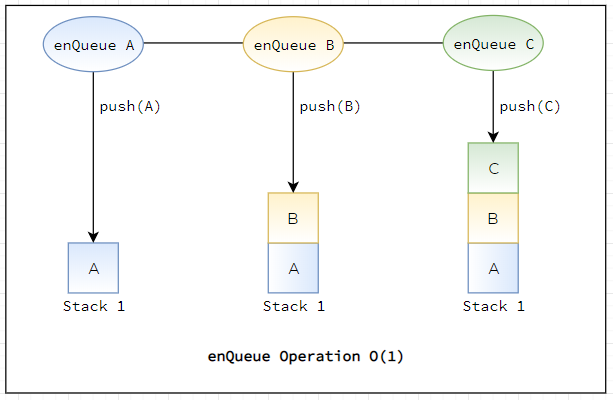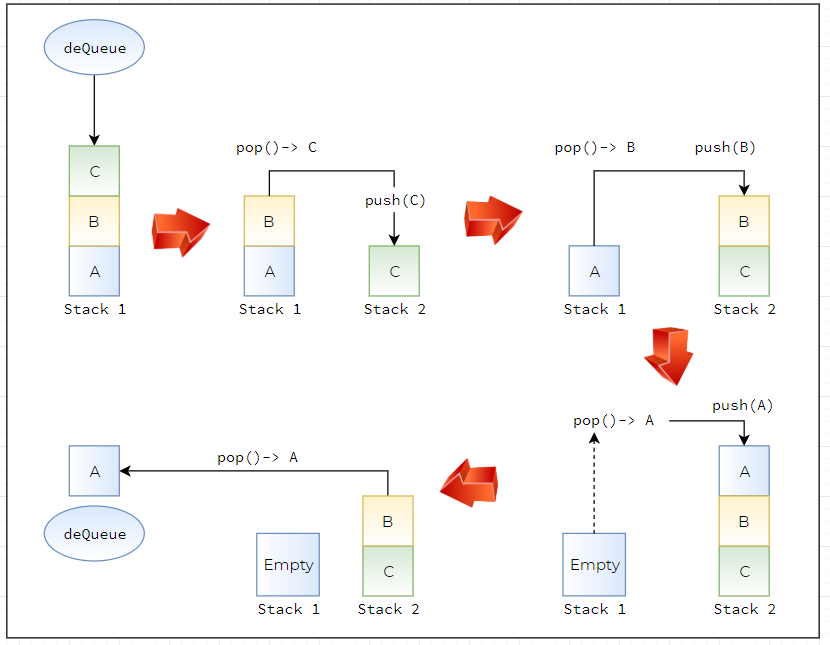## How can you implement a Queue using two stacks?

Implement the following operations of a queue using 2 stacks named `s1` and `s2`.

• push(x) → Push element x to the back of the `Queue`.
• pop() → Removes the element from in front of the `Queue`.
• peek() → Get the front element.
• empty() → Return whether the `Queue` is empty.

Notes:

• You must use only standard operations of a stack -- which means only `push to top``peek/pop from top``size`, and `is empty` operations are valid.
• Depending on your language, the stack may not be supported natively. You may simulate a stack by using a list or deque (double-ended queue), as long as you use only standard operations of a stack.
• You may assume that all operations are valid (for example, no pop or peek operations will be called on an empty queue).

The `enQueue `algorithm is very simple, Just push the value on the stack `s1`. The time complexity of `enQueue `operation is O(1).deQueue Algorithm

• If the stack `s2` is not empty then pop from `s2` and return the element.
• If the stack `s2` is empty, then transfer all elements from `s1` to `s2`. And return the popped element from `s2`. We can optimize the code a little by transferring only (n-1) elements from stack `s1` to stack `s2` and pop the nth element from stack `s2` return and the poped element.
• If stack s1 is also empty then throw an error.

The time complexity of this algorithm, if the stack `s2` is not empty then the complexity is O(1). But if the stack `s2` is empty, then we need to transfer all the elements from `s1` to `s2`. But if we carefully observe, the number of transferred elements and the number of popped elements from `s2` are equal. Due to this average complexity of pop operation, in this case, is O(1). The amortized complexity of pop operation is O(1). The following figure is the example of this algorithm.``````class MyQueue {

private Stack<Integer> enQueueStack;
private Stack<Integer> deQueueStack;

/** Initialize your data structure here. */
public MyQueue() {
enQueueStack = new Stack<>();
deQueueStack = new Stack<>();
}

/** Push element x to the back of queue. */
public void push(int x) {
enQueueStack.push(x);
}

/** Removes the element from in front of queue and returns that element. */
public int pop() {
Integer item = null;
if (!deQueueStack.isEmpty()) {
item = deQueueStack.pop();
} else {
while (!enQueueStack.isEmpty()) {
deQueueStack.push(enQueueStack.pop());
}
item = deQueueStack.pop();
}
return item;
}

/** Get the front element. */
public int peek() {
if (!deQueueStack.isEmpty()) {
return deQueueStack.peek();
} else {
while (!enQueueStack.isEmpty()) {
deQueueStack.push(enQueueStack.pop());
}
return deQueueStack.peek();
}
}

/** Returns whether the queue is empty. */
public boolean empty() {
return enQueueStack.isEmpty() && deQueueStack.isEmpty();
}
}``````

Amortized Analysis:

The amortized analysis gives the average performance (over time) of each operation in the worst case. The basic idea is that a worst-case operation can alter the state in such a way that the worst case cannot occur again for a long time, thus amortizing its cost. Consider this example where we start with an empty queue with the following sequence of operations applied:

`push1,push2,…,pushn,pop1,pop2…,popn `

The worst-case time complexity of a single pop operation is `O(n)`. Since we have `n` pop operations, using the worst-case per operation analysis gives us a total of `O(n2)` time. However, in a sequence of operations the worst case does not occur often in each operation - some operations may be cheap, some may be expensive. Therefore, a traditional worst-case per operation analysis can give overly pessimistic bound. For example, in a dynamic array, only some inserts take linear time, though others - a constant time. In the example above, the number of times pop operation can be called is limited by the number of push operations before it. Although a single pop operation could be expensive, it is expensive only once per `n` times (`Queue sSze`), when `s2` is empty and there is a need for data transfer between `s1` and `s2`. Hence the total time complexity of the sequence is :

`n (for push operations) + 2*n (for first pop operation) + n - 1 ( for pop operations) which is O(2*n).`

This gives `O(2n/2n) = O(1)` average time per operation.

Algorithm